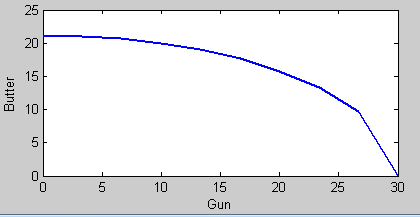# Suppose the production possibility frontier for guns (G) and better (B) is given by G^(2) +...

## Question:

Suppose the production possibility frontier for guns (G) and better (B) is given by {eq}G^{2} + 2B^{2} = 900 {/eq}. If you put G on x-axis, and B on y-axis, the slope of the tangent line at each point on PPF is {eq}\frac{-G}{2B} {/eq}.

a) Graph the production possibility frontier.

b) If individual's preference is U = G + 2B. How much B and G will be produced? Graph this allocation in the same graph as in part a.

## Production Possibility Frontier:

Production Possibility Frontier (PPF) is the combination of goods an economy can produce exhausting all resources it has at disposal. The PPF could be used to represent the level of efficiency within an economy.

## Answer and Explanation: 1

Become a Study.com member to unlock this answer!

a) The PPF is present below:b) The economy produces 17.3 units of gun and butter.

At the optimal output mix, the technical rate of...

See full answer below.